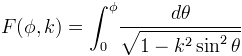Computes the incomplete elliptic integral of the first kind.

Namespace:  Meta.Numerics.Functions
Assembly:  Meta.Numerics (in Meta.Numerics.dll) Version: 4.1.4Syntax
```public static double EllipticF(
double phi,
double k
)```

#### Parameters

phi
Type: SystemDouble
k
Type: SystemDouble
The elliptic modulus, which must lie between zero and one.

#### Return Value

Type: Double
The value of the Legendre integral F(k).Remarks

Legendre's first incomplete elliptic integral is:When the integral angle is π, this function reduces to the complete elliptic integral of the first kind (EllipticK(Double).

Be aware that some authors use the the parameter m = k2 instead of the modulus k.See Also